##### Postgres SQRT() Function With Practical Examples

While working with Mathematical data, one of the most frequently performed tasks is finding the square root of a number. Postgres users may encounter a situation where they need to find a specific number's square root. Postgres provides a built-in SQRT() function to deal with such situations efficiently.

This post illustrates the Postgres SQRT() function and its working via practical examples.

How to Use SQRT() in PostgreSQL?

The SQRT() function accepts a positive numeric value and retrieves its square root. The below-given syntax is used to calculate the square root of a positive numeric value using the SQRT() function:

`SQRT(num);`

The “num” must be a positive value.

Example 1: Calculating the Square Root of a Positive Integers

The below snippet demonstrates the usage of the SQRT() function:

`SELECT SQRT(144);`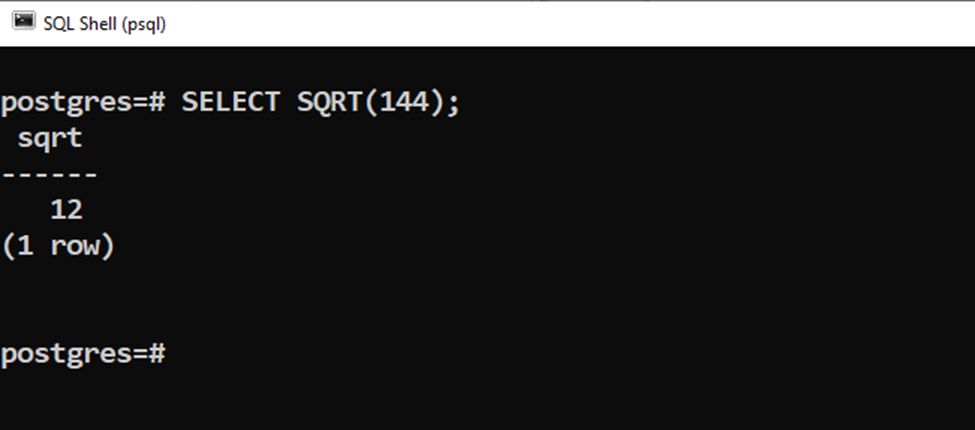The output shows that the square root of “144” is 12.

Example 2: Calculating the Square Root of a Positive Floating Point Value

Let’s learn how the SQRT() function work with floating point values:

`SELECT SQRT(145.22);`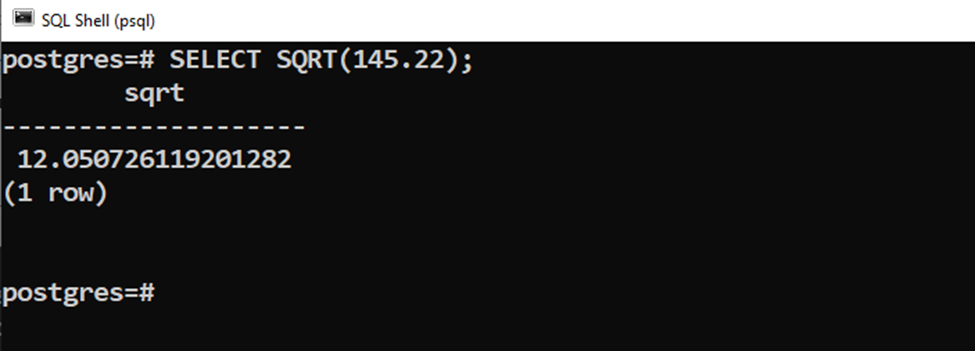The output shows that the square root of “145.22” is 12.05072.

Example 3: Calculating the Square Root of a Negative Numeric Value

Let’s pass a negative value to the SQRT() function and see how it deals with the negative values:

`SELECT SQRT(-145);`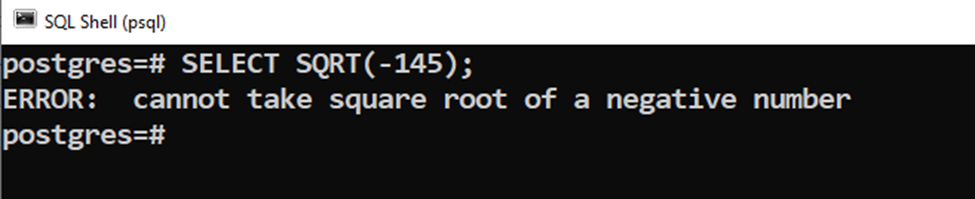The SQRT() function throws an error stating that it “can’t accept a negative value”.

Example 4: Finding the Square Root of the Table’s Data

Suppose we have a sample table that stores numeric data, as shown in the following snippet: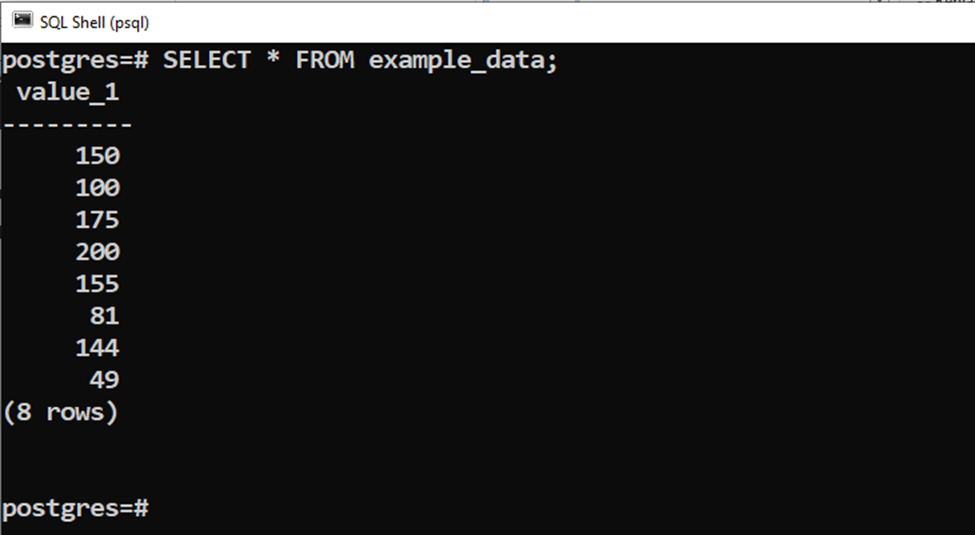The task is to find the square root of each entry. To achieve this particular task, we will utilize the following statement:

```SELECT Value_1, SQRT(value_1)
FROM example_data;```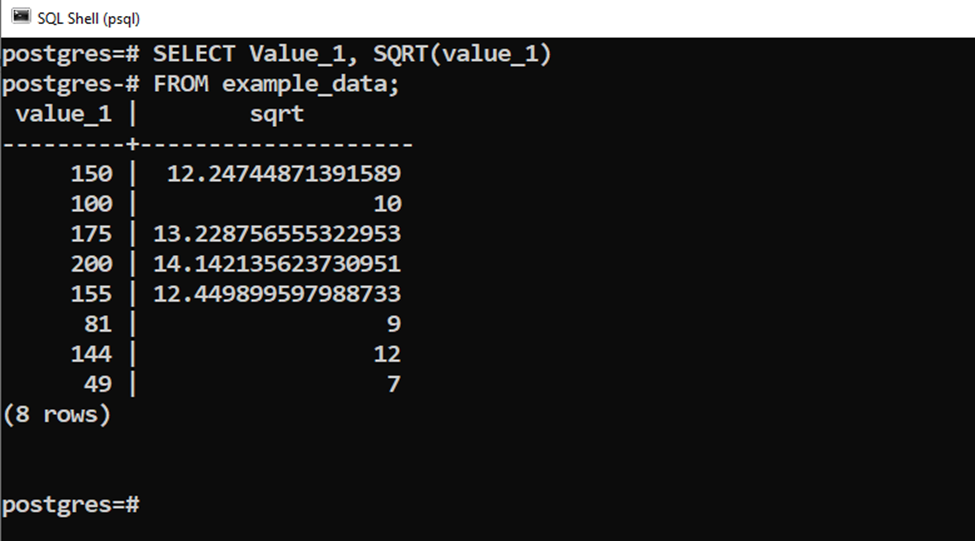This way, you can get the square root of the positive numbers using the Postgres SQRT() function.

Conclusion

The SQRT() is a built-in mathematical function that accepts a positive numeric value and retrieves its square root. It accepts only positive numbers, and passing negative values to the stated function results in an error. It can find the square root of any numeric value, such as fractional point values, integer values, etc. This blog post considered several use cases of the SQRT() function with practical examples.